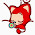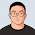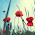### leetcode Question 133: Palindrome Partitioning II

Palindrome Partitioning II
Given a string s, partition s such that every substring of the partition is a palindrome.
Return the minimum cuts needed for a palindrome partitioning of s.
For example, given s = "aab",
Return 1 since the palindrome partitioning ["aa","b"] could be produced using 1 cut.
Analysis:
This is a DP problem! Also it is a double DP problem!
Why? Because, if we use the same algorithm in the Palindrome Partitioning I, definitely it will expire the time limit. When you are facing the problem asking "return the minimum/maximum, best, shortest...", it is also a good direction targeting the DP (sometimes greedy also works fine). If you are not familiar with DP problem, here is a very good reading from topcoder. Generally speaking, DP is not very easy, and most of the company interviews will NOT cover the DP, so don't worry too much.

For this problem, firstly we consider the main part.
It is a good way to look for the "state", and the "optimal solution" based on that state, to solve the DP problem. In other words, if you found the correct state, and the function of the state representing the optimal solution, all you need is some loops and initialization implementing the algorithm.

Here the state is not too hard ----  minimum cut.   Define res[i] = the minimum cut from 0 to i in the string.
The result w =e need eventually is res[s.size()-1].
We know res=0. Next we are looking for the optimal solution function f.
For example, let s = "leet".
f(0) = 0;  // minimum cut of str[0:0]="l", which is a palindrome, so not cut is needed.
f(1) = 1; // str[0:1]="le" How to get 1?
f(1) might be:  (1)   f(0)+1=1, the minimum cut before plus the current char.
(2)   0, if str[0:1] is a palindrome  (here "le" is not )
f(2) = 1; // str[0:2] = "lee" How to get 2?
f(2) might be:   (1)  f(1) + 1=2
(2)  0, if str[0:2] is a palindrome (here "lee" is not)
(3)  f(0) + 1,  if str[1:2] is a palindrome, yes!
f(3) = 2; // str[0:3] = "leet" How to get 2?
f(3) might be:   (1)  f(2) + 1=2
(2)  0, if str[0:3] is a palindrome (here "leet" is not)
(3)  f(0) + 1,  if str[1:3] is a palindrome (here "eet" is not)
(4)  f(1) + 1,  if str[2:e] is a palindrome (here "et" is not)
OK, output f(3) =2 as the result.

So, the optimal function is:

f(i) = min [  f(j)+1,  j=0..i-1   and str[j:i] is palindrome
0,   if str[0,i] is palindrome ]

The above algorithm works well for the smaller test cases, however for the big cases, it still cannot pass.
Why? The way we test the palindrome is time-consuming.

Also using the similar DP idea, we can construct the look-up table before the main part above, so that the palindrome testing becomes the looking up operation. The way we construct the table is also the idea of DP.
e.g.  mp[i][j]==true if str[i:j] is palindrome.
mp[i][i]=true;
mp[i][j] = true if str[i]==str[j] and (mp[i+1][j-1]==true or j-i<2 )  j-i<2 ensures the array boundary.

So, using this scheme to test the palindrome helps improve the efficiency and thus we can pass all the test cases. Details see the code below.

Code:
class Solution {
public:
int minCut(string s) {
// Start typing your C/C++ solution below
// DO NOT write int main() function
vector<int>res(s.size(),0);
bool mp[s.size()][s.size()];

for (int i=s.size()-1;i>=0;i--){
for (int j=i;j<s.size();j++){
if ((s[i]==s[j]) && (j-i<2 || mp[i+1][j-1])){
mp[i][j]=true;
}else{
mp[i][j]=false;
}
}
}

for (int i=0;i<s.size();i++){
int ms = s.size();
if (mp[i]){
res[i]=0;
}else{
for (int j=0;j<i;j++){
if (mp[j+1][i] && ms>res[j]+1 ) {
ms=res[j]+1;
}
}
res[i]=ms;
}
}
return res[s.size()-1];
}
};


1.2.Excellent explanation, easy and elegant

3.有个更好的算法，只需要O(n)空间：https://oj.leetcode.com/discuss/9476/solution-does-not-need-table-palindrome-right-uses-only-space.
其实这题关键在于怎样判断palindrome，而判断的方法有两种：一种是递归构造，另一种是枚举所有中心点然后向两方向扩散。我发现绝大多数解法都是基于第一种的，但其实如果基于第二种的话能够省去那个O(n^2)的空间，这也就是链接中方法的思想。﻿

4.不太明白 line 25 为什么要 ms>res[j]+1

可不可以在初始化res的时候, 赋值INT_MAX
然后每次res[i] = min(res[i], 1+res[j]);

5.This comment has been removed by the author.

6.awesome explanation! Great job!

7.nice explained!